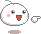## R.S Aggarwal Quantitative Aptitude PDF

Hello friends, Today we are sharing a very important and easy PDF of R.S. Agarwal Quantitative Aptitude PDF. We have also included some of the most important questions related to Quantitative Aptitude in the PDF for your better preparation for all the government exams(U.P.P, UPSI,UPTGT, PGT,UPTET/CTET, HTET, RTET, UDA/LDA, RO/ARO, BEd, LLB, RRB, सचिवालय, अस्सिस्टेंट ग्रेड, ग्राम पंचयत अधिकारी, स्टेनोग्राफर, लेखा परीक्षक, हिनीद अनुवादक परीक्षा, डिप्टी जेलर, बैंक परीक्षा ,एल आई सी, लेखपाल इत्यादि ). If you are preparing for your exams in the last few days then this R.S. Aggarwal Quantitative Aptitude PDF is very important for you.

There are around 20-25 questions in each Government Exams related to R.S. Aggarwal Quantitative Aptitude PDF and you can solve 18-20 questions out of them very easily by reading these Notes of Quantitative Aptitude. The complete PDF of R.S. Aggarwal Quantitative Aptitude is attached below for your reference, which you can download by clicking at the Download Button.

If you have any doubt or suggestion regarding the PDF then you can tell us in the Comment Section given below, we will be happy to help you. We wish you a better future.

## Topics Included in the PDF

• NUMBERS
• Place Value or Local Value of a Digit in a Numeral
• Face Value
• Natural Numbers
• Whole Numbers
• Integers
• Even Numbers
• Odd Numbers
• Prime Numbers
• Composite Numbers
• Co-primes
• TESTS OF DIVISIBILITY
• MULTIPLICATION BY SHORT CUT METHODS
• BASIC FORMULAE
• DIVISION ALGORITHM OR EUCLIDEAN ALGORITHM
• Arithmetic Progression (A.P.)
• Geometrical Progression (G.P.)
• H.C.F.
• L.C.M.
• DECIMAL FRACTIONS
• SIMPLIFICATION
• SQUARE ROOTS AND CUBE ROOTS
• AVERAGE
• PROBLEMS ON NUMBERS
• PROBLEMS ON AGES
• SURDS AND INDICES
• PERCENTAGE
• IMPORTANT FACTS
• RATIO AND PROPORTION
• PARTNERSHIP
• CHAIN RULE
• TIME AND WORK
• PIPES AND CISTERNS
• TIME AND DISTANCE
• PROBLEMS ON TRAINS

#### # We have explained some of the topics given in the PDF below:-

1.NUMBERS:-

1. Numeral: In Hindu Arabic system, we use ten symbols 0, 1, 2, 3, 4, 5, 6, 7, 8, 9 called digits to represent any number.
2. Natural Numbers: Counting numbers 1, 2, 3, 4, 5,….. are called natural
numbers.
3. Whole Numbers: All counting numbers together with zero form the set of whole
numbers.
4. Integers : All natural numbers, 0 and negatives of counting numbers i.e.,
{…, – 3 , – 2 , – 1 , 0, 1, 2, 3,…..} together form the set of integers.
5. Even Numbers: A number divisible by 2 is called an even number, e.g., 2, 4, 6, 8,
10, etc.
6. Odd Numbers: A number not divisible by 2 is called an odd number. e.g., 1, 3, 5, 7,
9, 11, etc.
7. Prime Numbers: A number greater than 1 is called a prime number if it has exactly
two factors, namely 1 and the number itself.
8. Composite Numbers: Numbers greater than 1 which are not prime, are known as
composite numbers, e.g., 4, 6, 8, 9, 10, 12.
9. Co-primes: Two numbers a and b are said to be co-primes if their H.C.F. is 1. e.g.,
(2, 3), (4, 5), (7, 9), (8, 11), etc. are co-primes,
10. Arithmetic Progression (A.P.): If each term of a progression differs from its
preceding term by a constant, then such a progression is called an arithmetical
progression.
11. Geometrical Progression (G.P.): A progression of numbers in which every term bears a constant ratio with its preceding term, is called a geometrical progression.
12. Factors and Multiples: If a number a divides another number b exactly, we say that a
is a factor of b. In this case, b is called a multiple of a.
13. Highest Common Factor (H.C.F.) or Greatest Common Measure (G.C.M.) or
Greatest Common Divisor (G.C.D.): The H.C.F. of two or more than two numbers is
the greatest number that divides each of them exactly.
14. Least Common Multiple (L.C.M.): The least number which is exactly divisible by
each one of the given numbers is called their L.C.M.
15. Decimal Fractions: Fractions in which denominators are powers of 10 are known as decimal
fractions.
16. Conversion of a Decimal Into Vulgar Fraction: Put 1 in the denominator under the decimal
point and annex with it as many zeros as is the number of digits after the decimal point.
17. Addition and Subtraction of Decimal Fractions: The given numbers are so
placed under each other that the decimal points lie in one column
18. Multiplication of a Decimal Fraction By a Power of 10: Shift the decimal
point to the right by as many places as is the power of 10.
19. Multiplication of Decimal Fractions: Multiply the given numbers considering
them without the decimal point.
20. Dividing a Decimal Fraction By a Counting Number: Divide the given
number without considering the decimal point, by the given counting.
Related Posts:-Average questions in maths SSCMaths pervious year PaperAdvanced math for ssc pdfMaths Notes pdfAdvance Math for SSC CGL

## R.S. Agarwal Quantitative Aptitude PDF

 Book Name: R.S. Aggarwal Quantitative Aptitude PDF Quality: Excellent Format: PDF Size: 2.55 MB Pages: 194 Page Language: english

MyNotesAdda.com will update many more new pdf and study materials and exam updates, keep Visiting and share our post, So more people will get this.

The above PDF is only provided to you by MyNotesAdda.com we are not the makers of the PDF, if you like the PDF or if you have any kind of doubt, suggestion or query regarding the same, feel free to contact us at our Mail id- [email protected] or you can chat with us in the comment section given below.